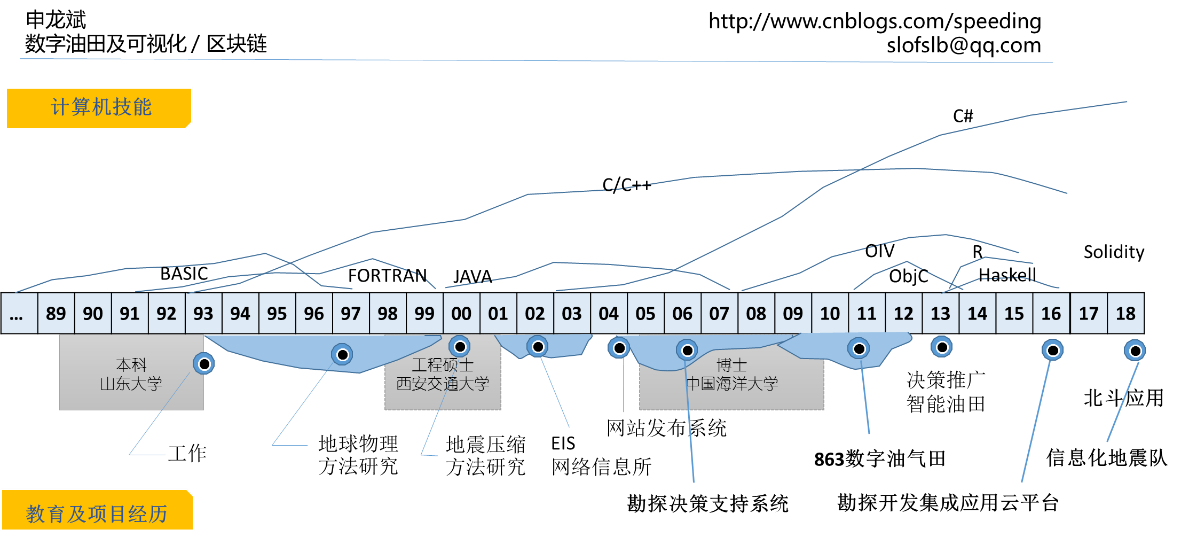# 通过欧拉计划学Rust编程（第500题）

“欧拉计划”的网址： https://projecteuler.net

https://projecteuler.net/problem=500

120的因子个数为16，事实上120是最小的有16个因子的数。

fn min_number_has_factors(x: u64) -> u64 {
for n in 2.. {
let groups = factors_group(n);
let factors_num = groups.iter().map(|(_, x)| x + 1).product::<u64>();
if factors_num == x {
println!("{}, divisors num: {}", n, factors_num);
print_factors_group(groups);
return n;
}
}
0
}

// 如果一个数有这些因子：[2, 2, 3, 3, 3, 3, 5, 7]
// 则得到：[(2,2), (3,4), (5,1), (7,1)]
fn factors_group(n: u64) -> Vec<(u64, u64)> {
let factors = primes::factors(n);
let groups = factors
.iter()
.group_by(|e| **e)
.into_iter()
.map(|(k, group)| (k, group.count() as u64))
.collect::<Vec<(u64, u64)>>();
groups
}

fn print_factors_group(groups: Vec<(u64, u64)>) {
println!(
"{}",
&groups
.iter()
.map(|(k, v)| k.to_string() + &"^" + &v.to_string())
.join(" * ")
);
println!(
"divisors num:  {}",
&groups
.iter()
.map(|(_, v)| "(".to_string() + &v.to_string() + &"+1)")
.join(" * ")
);
}


min_number_has_factors(4); // 2^2
min_number_has_factors(8); // 2^3
min_number_has_factors(16); // 2^4
min_number_has_factors(32); // 2^5
min_number_has_factors(64); // 2^6
min_number_has_factors(128); // 2^7
min_number_has_factors(256); // 2^8


6 = 2^1 * 3^1

24 = 2^3 * 3^1

120 = 2^3 * 3^1 * 5^1

840 = 2^3 * 3^1 * 5^1 * 7^1

7560 = 2^3 * 3^3 * 5^1 * 7^1

83160 = 2^3 * 3^3 * 5^1 * 7^1 * 11^1

1081080 = 2^3 * 3^3 * 5^1 * 7^1 * 11^1 * 13^1因子个数 2 =  (2^1)
[b0] = 

[b0,b1] = [1,1]

[b0,b1] = [2,1]

[b0,b1,b2] = [2,1,1]

[b0,b1,b2] = [2,2,1]

[b0,b1,b2,b3] = [2,2,1,1]

[b0,b1,b2,b3,b4] = [2,2,1,1,1]

[b0,b1,b2,b3,b4,b5] = [2,2,1,1,1,1]fn p500(n: usize) -> u64 {
let mut pset = PrimeSet::new();
let primes: Vec<_> = pset.iter().take(n).collect();
let primes_log: Vec<_> = primes.iter().map(|x| (*x as f64).log10()).collect();

let mut b = vec!;
for _i in 2..=n {
let mut min = primes_log[b.len()];
let mut pos = b.len(); // 默认尾部增加一个
for j in 0..b.len() {
let temp = 2_f64.powf(b[j] as f64) * primes_log[j];
if temp < min {
pos = j;
min = temp;
}
if b[j] == 1 {
break; // 后面的都不用判断了
}
}
if pos == b.len() {
b.push(1);
} else {
b[pos] += 1;
}
}

let mut result = 1_u64;
for i in 0..b.len() {
let exp = 2_u32.pow(b[i]) - 1;
for _j in 0..exp {
result = result * primes[i] % 500500507;
}
}
result
}


--- END ---

----==== Email: slofslb (GTD) qq.com 请将(GTD)换成@ ====----

---- 魔方桥牌象棋、游戏人生...